# 17.33. プロセッシングでRスクリプトを使用する¶

Module contributed by Matteo Ghetta - funded by Scuola Superiore Sant'Anna

Processing (with the `Processing R Provider` plugin) makes it possible to write and run R scripts inside QGIS.

R has to be installed on your computer and the PATH has to be correctly set up. Moreover Processing just calls the external R packages, it is not able to install them. So be sure to install external packages directly in R. See the related chapter in the user manual.

If you have package problems, it may be related to missing mandatory packages required by Processing, like `sp`, `rgdal` and `raster`.

## 17.33.1. スクリプトを追加する¶

Adding a script is simple. The easiest way is to open the Processing toolbox and choose Create new R script... from the R menu (labelled with an R icon) at the top of the Processing Toolbox. You can also create the script in for instance a text editor and save it in your R scripts folder (`processing/rscripts`). When it has been saved there, it will be available for editing by right-clicking on the script name in the processing toolbox and then choose Edit Script...).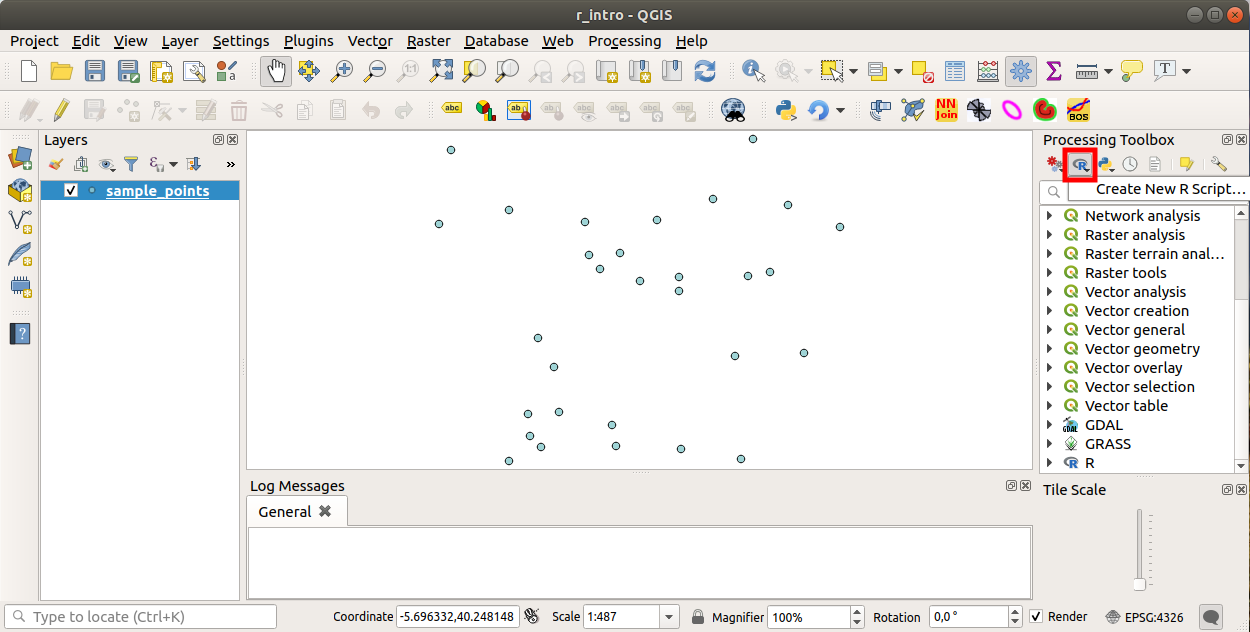プロセッシングの中にRが見あたらない場合は、 プロセッシング ▶ オプション ▶ プロバイダー を有効にする必要があります

スクリプト本体を追加できる前にいくつかのパラメーターを指定する必要がある スクリプトエディタウィンドウ を開きます。

## 17.33.2. プロットを作成する¶

このチュートリアルでは、ベクターレイヤーフィールドの 箱ひげ図 を作成しようとしています。

`exercise_data/processing/r_intro/` フォルダの下にある `r_intro.qgs` QGISプロジェクトを開きます。

### 17.33.2.1. スクリプトのパラメーター¶

エディタを開いて、それの最初に書き始めます。

スクリプト本体の にいくつかのパラメーターを指定する 必要があります

1. The name of the group (plots in this case) in which you want to put your script (if the group does not exist, it will be created):

```##plots=group
```

You will find your script in the plots R group in the Processing toolbox.

2. You have to tell Processing that you want to display a plot (in this example):

```##showplots
```

You will then find a link to the plot in the Result Viewer panel (can be turned on / off in View ▶ Panels and with Processing ▶ Results Viewer).

3. You also need to tell Processing about your input data. In this example we want to create a plot from a field of a vector layer:

```##Layer=vector
```

Processing now knows that the input is a vector. The name Layer is not important, what matters is the vector parameter.

4. Finally, you have to specify the input field of the vector layer (using the name you have provided above - Layer):

```##X=Field Layer
```

Processing now knows that you need a field of Layer, and that you will call it X.

5. It is also possible to define the name of your script using `name`:

```##My box plot script=name
```

If not defined, the file name will be used as the name of the script.

### 17.33.2.2. スクリプト本体¶

```boxplot(Layer[[X]])
```

boxplot is the name of the R function, the parameter Layer is the name that you have defined for the input dataset and X is the name you have defined for the field of that dataset.

The parameter X has to be within double square brackets (`[[]]`).

```##Vector processing=group
##showplots
##Layer=vector
##X=Field Layer
boxplot(Layer[[X]])
```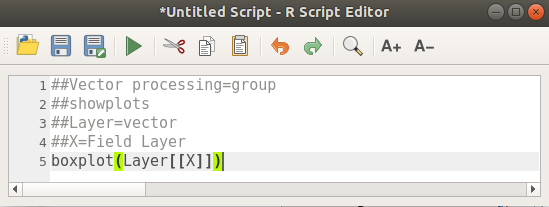Save the script in the default path suggested by Processing (processing/rscripts). If you have not defined a `name` in the script heading, the file name you choose will become the name of the script in the Processing toolbox.

You can save the script wherever you like, but Processing will then not be able to include it in the processing toolbox automatically, so you have to upload it manually.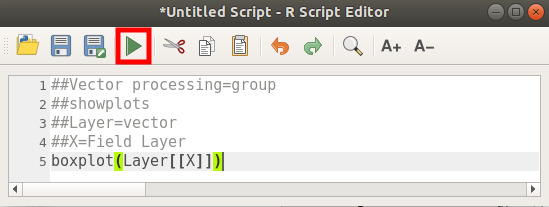Once the editor window has been closed, use the text box of Processing to find your script: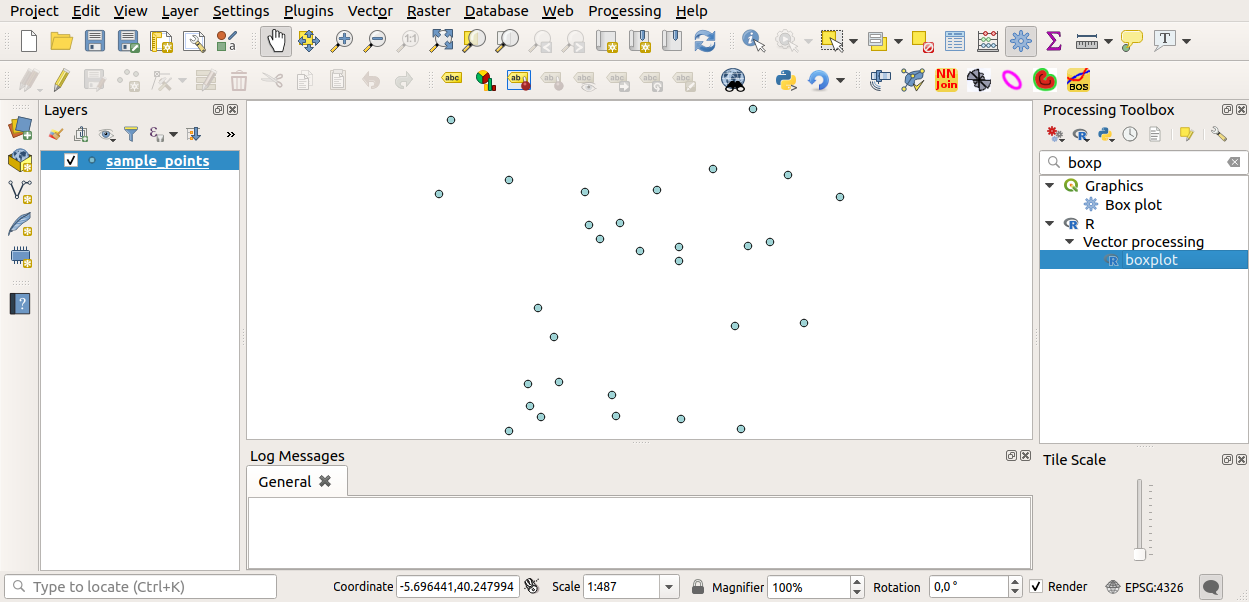You can now fill the parameters required in the Processing algorithm window:

• for Layer choose sample_points

• for the X field choose value

[実行] をクリックしてください。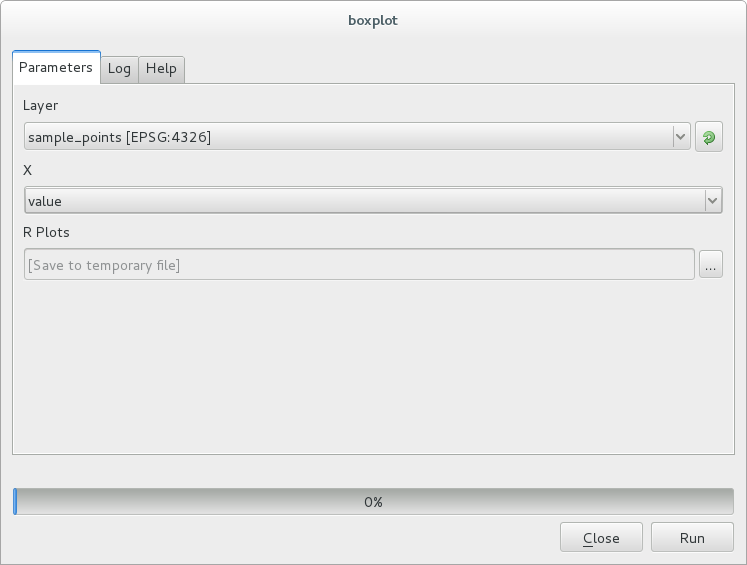Click on the link in the viewer and you will see: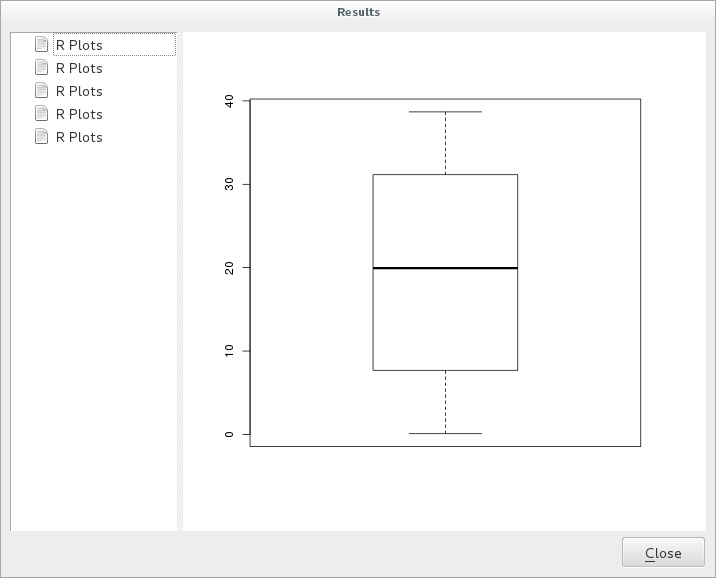You can open, copy and save the image by right clicking on the plot.

## 17.33.3. ベクターを作成する¶

You can also create a vector layer and have it automatically loaded into QGIS.

The following example has been taken from the `Random sampling grid` script that can be found in the online collection of R scripts (the scripts in this online collection can be found in https://github.com/qgis/QGIS-Processing/tree/master/rscripts).

The aim of this exercise is to create a random point vector layer using an input vector layer to restrict the extent using the `spsample` function of the `sp` package.

### 17.33.3.1. スクリプトのパラメーター¶

1. Specify the name of the group in which you want to put your script, in this case Point pattern analysis:

```##Point pattern analysis=group
```
2. Define an input parameter (a vector layer) that will constrain the placement of the random points:

```##Layer=vector
```
3. Set an input parameter for the number of points that are going to be created (`Size`, with a default value of `10`):

```##Size=number 10
```

注釈

Since a default value (`10`) is defined, the user can change this number or can leave the parameter without a number.

4. Specify that there is an output vector layer (called `Output`):

```##Output=output vector
```

### 17.33.3.2. スクリプト本体¶

1. Use the `spsample` function:

```pts=spsample(Layer, Size, type="random")
```

The function uses the Layer to constrain the placement of the points (if it is a line layer, a points will have to be on one of the lines in the layer, if it is a polygon layer, a point will have to be within a polygon). The number of points is taken from the Size parameter. The sampling method is random.

2. Generate the output (the `Output` parameter):

```Output=SpatialPointsDataFrame(pts, as.data.frame(pts))
```

```##Point pattern analysis=group
##Layer=vector
##Size=number 10
##Output=output vector
pts=spsample(Layer, Size, type="random")
Output=SpatialPointsDataFrame(pts, as.data.frame(pts))
```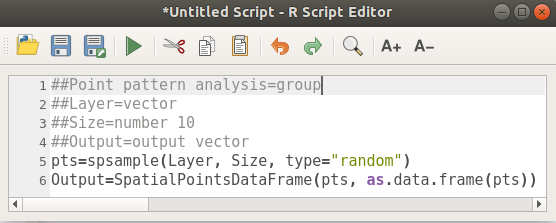Save it and run it, clicking on the run button.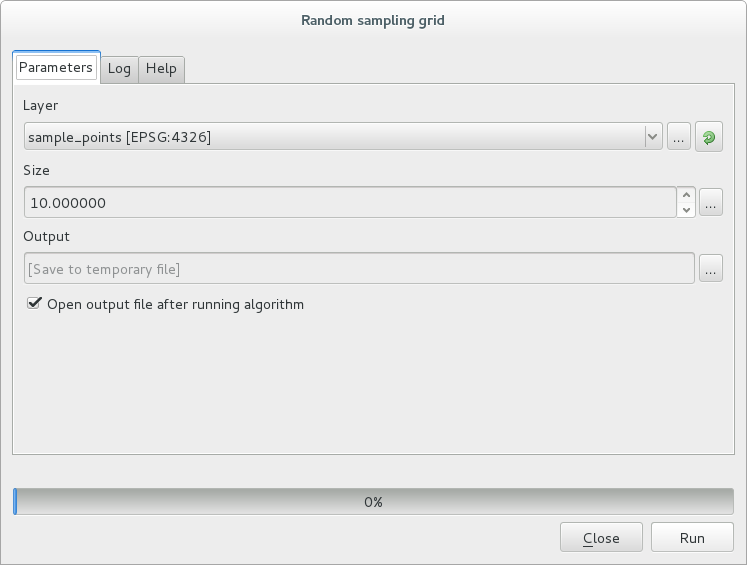そして実行をクリックしてください。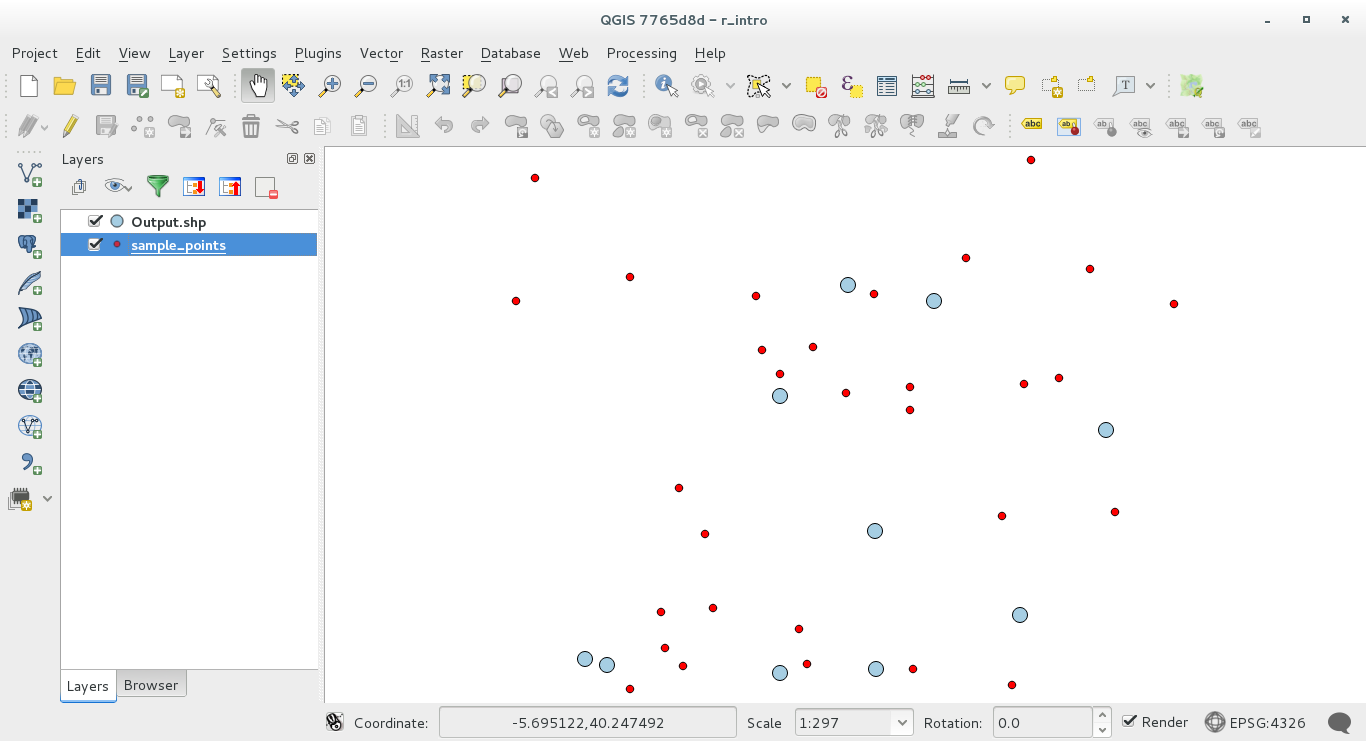Processing (with the `Processing R Provider plugin`) uses special syntax to get the results out of R:
• コマンドの前に `>` は、 `>lillie.test(Layer[[Field]])` 中でのように、結果がR出力（結果ビューア）に送られるべきことを意味します
• `+` after a plot enables overlay plots. For example ```plot(Layer[[X]], Layer[[Y]]) + abline(h=mean(Layer[[X]]))```